# Using Interferometer Stabilization for Photonics and Imaging

This article outlines how to measure, control, and stabilize the relative distance of two interferometer paths to sub-wavelength precision over numerous wavelengths, using the Zurich Instruments MFLI – a 500 kHz/5MHz Lock-in Amplifier.

On one of the arms of the interferometer, a phase modulation is introduced. This generates a signal in order to display the relative displacement both continuously and linearly. An internal PID controller then processes this signal, in order to stabilize the relative path length to an adjustable set-point.

## Motivation

Optical interferometers perform a precise measurement of the relative phase difference between two paths of light propagation. As used in quantitative phase imaging, one application constitutes the detection and subsequent control of relative optical path length changes of two interferometer arms to a fraction of the light wavelength . The Michelson-Morley interferometry scheme is depicted in Figure 1. However, other configurations such as the Mach-Zehnder are also commonly used.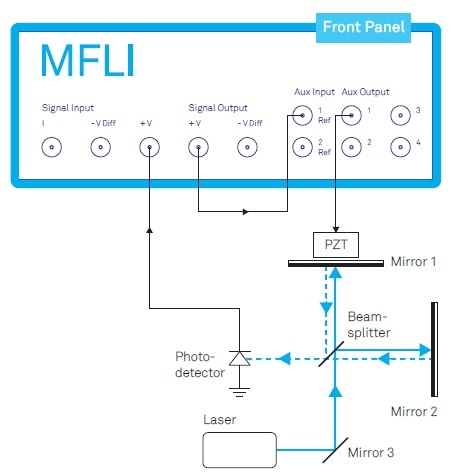Figure 1. Michelson-Morley interferometer actively stabilized by a closed-loop system using the Zurich Instruments MFLI Lock-in Amplifier. The Auxiliary Output provides both a modulating signal and a controlling offset for the piezo electric transducer (PZT). The external loop-back from the Signal Output to the Auxiliary Input is required to generate the linearized and continuous error signal for PID control (see text).

Changes in air pressure or temperature can cause miniscule relative changes in the two pathways’ refractive index. This leads to a large measurable signal. The high sensitivity has its downsides – for instance, factors such as variations in temperature gradients or mechanical noise can introduce small fluctuations. These can cause miniscule mirror displacements, which can induce systematic measurement errors.

Such error sources can be suppressed by active stabilization and continuous measurement of the relative interferometer phase angle. Yet, by directly using the relative phase angle as an input for a feedback system, the control range is limited to less than π/2 or a quarter of the wavelength. There are also other downsides.

In this article an elegant technique is introduced in  which is based on the work presented in . This technique offers the following advantages:

• The ability to work at arbitrary relative phase angles
• The ability to cover an arbitrary large displacement range
• It is simple to set up and monitor
• Operation can be controlled either via a computer or manually.

## Description

The example shown in Figure 1 demonstrates a light intensity I detected by the photodetector which satisfies the following expression: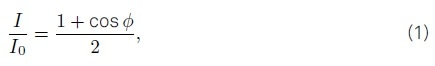where I0 is the laser light’s at wavelength λ, and ϕ is the phase difference between the interferometer’s two arms.

The photodetector signal which is described in Equation 1 is presented in Figure 2 (a). Two challenges faced when using the photodetector signal to detect the mirror displacement via the ϕ phase are shown. One of these is represented by the variation in sensitivity of the points of maximum slope and the curves extrema. The other is represented by the difficulty of getting rid of the ambiguity which is imposed by the photodetector signal I as a non-monotone function of ϕ.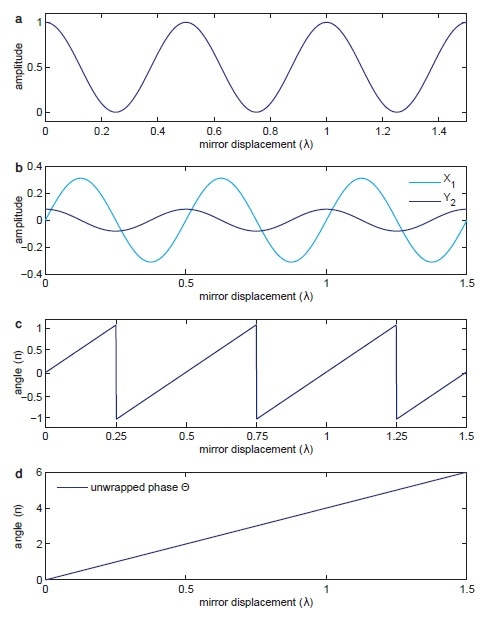Figure 2. (a) shows the sinusoidal signal detected by the photodetector over the relative displacement between mirrors 1 and 2. (b) shows the signals X1 and Y2 demodulated at Ω and 2Ω. (c) shows the phase angle between the normalized X1 and Y2 components given in Equation 5. (d) shows the unwrapped version of the same phase in (c) used by the PID controller to drive the PZT.

It is not possible to see whether the displacement is continuing in the same direction or going through a turning point when one is reading the photodetector voltage at the extrema points. Both of the above issues can be resolved via the introduction of a phase modulation to one of the beam paths. This can be achieved by applying a sinusoidal voltage of frequency Ω to the piezoelectric transducer, which subsequently controls the relative path difference.

This phase modulation can be described as the following: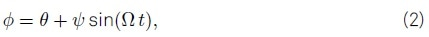where ψ represents the modulation depth and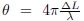is the initial phase offset due to the length difference ΔL between the arms of the interferometer. By substituting Equation 2 for ϕ in Equation 1, a phase-modulated signal which consists of higher harmonics of Ω results.

With the use of the Jacobi-Anger expansion, the first and the second harmonics, I1 and I2, can be expressed in terms of Bessel functions J1 and J θ at the modulation depth ψ as shown below.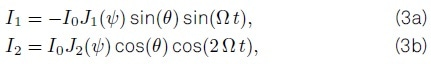Where the components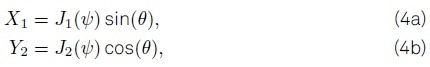resemble the form of the first and the second derivatives of the original fringe pattern. Illustrated in Figure 3 shows how the slowly-varying signal components X1 and Y2, which are depicted in Figure 2 (b), are extracted from the original photodetector signal by demodulation at the frequencies Ω and 2Ω.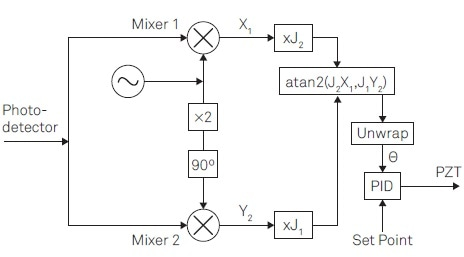Figure 3. Linear phase control for interferometer stabilization requires the following steps: (1) Provide a reference signal at Ω to apply a phase modulation. (2) Apply lock-in detection to the photodetector signal to obtain the demodulation signalsX1 and Y2 at the modulation frequency Ω and its 2nd harmonic. (3) Apply correction factors J1 and J2. (4) Determine the phase θ by atan2(J2X1; J1Y2) (5) Unwrap the phase θ. (6) Apply PID controller to provide feedback.

In order for the response signal to be linearized, it is vital that both components X1 and Y2 contribute with precisely the same strength. This is achieved by either adjusting the modulation depth to ψ = 2.63 rad where J1(ψ) and J2(ψ) are equal, or by introducing the correction factors J1 and J2, as indicated in Figure 3. In both cases, the resulting parametric plot of these two signals should describe a circle instead of an ellipse. This way, the phase offset θ is obtained by the following expression: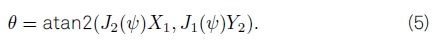It is worth noting that the function ‘atan2’ is used to unambiguously determine the phase within a range -π < θ ≤ π from the two arguments. Figure 2 (c) shows the result as compared with the original photodetector signal.

In order to extend the available phase range from the ‘atan2’ operation to an infinite scale, a phase unwrap is performed as a final step for obtaining a monotonic function of displacement between the arms. Figure 2 (d) illustrates the resulting unwrapped phase signal against the relative displacement in units of wavelength.

In order to provide feedback to the PZT in the form of an offset voltage, the signal is now able to be used by a PID controller. This controls the interferometer’s relative path lengths, and keeps the phase precisely at the defined set-point of the PID controller. At this point, a direct measure of the unintended and intended setup disturbances is delivered by the remaining error signal of the closed-loop controller.

The modulation in one of the arms of the interferometer is a pivotal aspect of this scheme. A clear limitation of this is that there is only an average, rather than an absolute, stabilization. Nevertheless, a proper synchronization with the repetition rate of the pulsed laser can remove this issue for pulsed laser experiments where the stabilization is performed using a CW laser.

## Implementation

The mechanical resonance frequency of the piezo-mirror assembly shown in Figure 1 is, in practice, a few ten kHz at most. This imposes an upper limit on the modulation speed as well as the bandwidth of the loop filter. Modulation bandwidth of as high as 200 kHz can be handled by the MFLI, which is limited by the Auxiliary Output bandwidth. The bandwidth of the photodetector can be as high as 5 MHz. The setup properties determine the maximum loop filter bandwidth, which can be as high as 50 kHz.

The MFLI must be equipped with the MF-MD Multi-demodulation and the MF-PID Quad PID/PLL Controller options, in order to implement the scheme shown in Figure 3. In order to determine the phase between the output of two independent demodulators and apply the correction coefficients J1(ψ) and J2(ψ) an intriguing technique is employed, which is illustrated in Figure 4.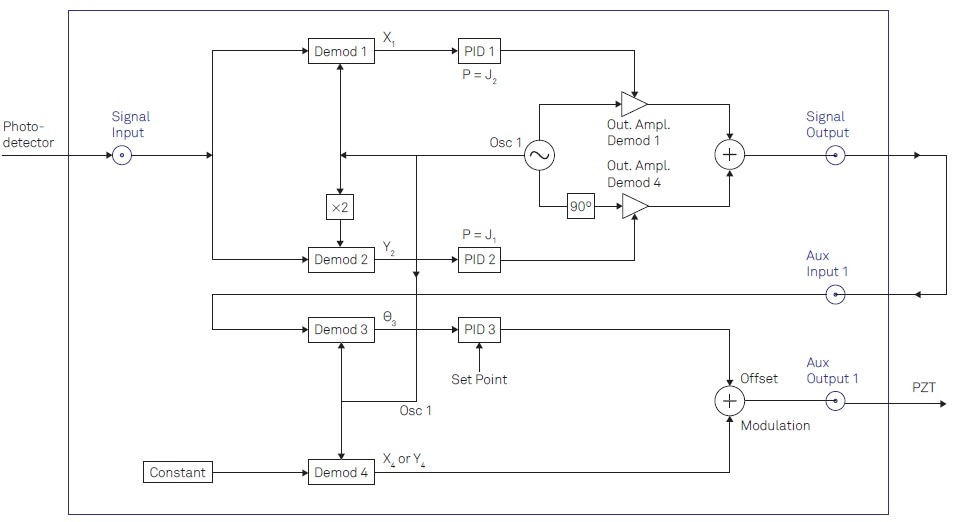Figure 4. Detailed description of the instrument configuration to be implemented using the LabOne user interface. In total, 4 demodulators (Demods) of the instruments are required. Two PID controllers are used as proportionate functions to apply the Bessel coefficients. The third PID controller is used to drive the offset signal controlling the mirror displacement.

The path of the demodulator (Demod) 4 is used to generate the reference signal at frequency Ω, which is sent out via the Auxiliary Output in order to drive the PZT. Numerous harmonics of Ω are included in the photodetector signal, as a result of the phase modulation. The photodetector signal is received at the Signal Input and demodulated at Ω by Demod 1 in order to obtain X1 and 2Ω by Demod 2 in order to get Y2, as depicted in Figure 4.

PID controllers 1 and 2 are used in their proportionate mode, in order to apply the correction factors J1(ψ) and J2(ψ) to the demodulated signals X1 and Y2 (i.e. the I and D parts are set at zero). The amplitude of a sine and a cosine wave is then altered at Ω through the path of Demod 1 and Demod 4, using the PID outputs. The signal which results, and which is available at the Signal Output, is expressed as follows: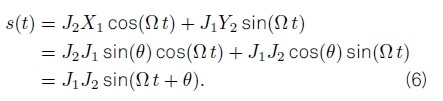The information in Figure 6 suggests that the phase difference θ , which is a result of the length difference between the interferometer’s two arms, is now able to be extracted by demodulating s(t) at frequency Ω. This is achieved by demodulating the signal using Demod 3, after the Signal Output has been looped back to the Auxiliary Input, as demonstrated in Figure 4.

Depicted in Figure 2 (c), the phase extracted by Demod 3 is described by Equation 5. The phase signal is subsequently used as an input for PID 3, which compares the signal to the set-point after unwrapping the phase. Once it has passed through the PID controller, the loop is closed as the signal is added to the reference signal in the Auxiliary Output connected to the PZT.

LabOne, the control software of Zurich Instruments, delivers a web-based user interface in order to set this up conveniently. It has a lot of powerful tools for further analysis. A primary step, for instance, could involve characterizing the transfer function of the PZT-mirror assembly by recording a Bode plot using the Parametric Sweeper tool. An approximate indication of the maximum loop filter bandwidth is provided the frequency at which the phase nears ±90°.

One could display the two signals X1 and Y2 in the integrated FFT Spectrum Analyzer in the second step, in order to confirm the signal dynamics. It is possible to easily derive the gain parameters for PID 3 from the PID Adviser, once a proper estimate for the required loop filter bandwidth is obtained. This can then be iteratively refined. It is also possible to entirely automate this entire procedure with the use of any of the LabOne APIs, which are available for LabVIEW, MATLAB, .NET, Python, and C, in order to integrate it into an existing control environment.

## Fast Modulation

Setups which require high-speed modulators, such as electro-optical modulators (EOM) or acousto-optical modulators (AOM), are able to operate at modulation frequencies of several hundred MHz. This enables noise components at higher frequencies to be canceled out by higher loop-filter bandwidths.

The Zurich Instruments UHFLI is recommended for these cases. It is a 600 MHz Lock-in Amplifier, which it is also possible to equip with 4 PID controllers in order to implement the scheme outlined above. The primary difference in implementation with the UHFLI is that it has the ability to generate the reference signal at a Signal Output rather than an Auxiliary Output.

The modulation frequency’s limitation to the Auxiliary Output bandwidth is eliminated, enabling the reference signal to cover the instrument’s entire frequency range – in this case, as high as 600 MHz.

The other Signal Output is used for the signal s(t) which contains the phase information. This is once more looped back to one of the Signal Inputs in order to extract its phase. The photodetector signal is received by the other Signal Input, and is demodulated at the first and second harmonics.

In order to freely select numerous demodulators, assign them to appropriate signals, and adjust their frequency and phase, it is necessary to use the UHF-MF Multi-frequency option. It is also necessary to use three of the four PID controllers which are provided by the UHF-PID Quad PID/PLL Controller option, in order to apply the correction factors and generate the control signal for mirror displacement.

## Conclusion

Zurich Instruments provides single-instrument solutions for interferometer stabilization and control up to modulation frequencies as high as 600 MHz, represented by the UHFLI and the MFLI. The LabOne user interface can be used to easily and rapidly implement the scheme presented above, as it has numerous built-in tools which are able to monitor and characterize the experimental setup. With the use of LabOne APIs, full computer control is also available.

## References

 H. Iwai, C. Fang-Yen, G. Popescu, A. Wax, K. Badizadegan, R. R. Dasari, and M. S. Feld. Quantitative phase imaging using actively stabilized phase-shifting low-coherence interferometry. Opt. Lett., 29(20):2399–2401, 2004.

 A. A. Freschi and J. Frejlich. Adjustable phase control in stabilized interferometry. Opt. Lett., 20(6):635–637, 1995.

 U. Minoni, E. Sardini, E. Gelmini, F. Docchio, and D. Marioli. Adjustable phase control in stabilized interferometry. Rev. Sci. Instrum., 62:2579, 1991.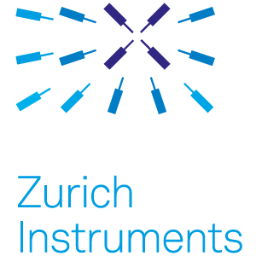This information has been sourced, reviewed and adapted from materials provided by Zurich Instruments.

## Citations

• APA

Zurich Instruments AG. (2019, March 28). Using Interferometer Stabilization for Photonics and Imaging. AZoNano. Retrieved on August 08, 2020 from https://www.azonano.com/article.aspx?ArticleID=5174.

• MLA

Zurich Instruments AG. "Using Interferometer Stabilization for Photonics and Imaging". AZoNano. 08 August 2020. <https://www.azonano.com/article.aspx?ArticleID=5174>.

• Chicago

Zurich Instruments AG. "Using Interferometer Stabilization for Photonics and Imaging". AZoNano. https://www.azonano.com/article.aspx?ArticleID=5174. (accessed August 08, 2020).

• Harvard

Zurich Instruments AG. 2019. Using Interferometer Stabilization for Photonics and Imaging. AZoNano, viewed 08 August 2020, https://www.azonano.com/article.aspx?ArticleID=5174.